QQ:1090930740# 广东省江门市紫茶小学人教版数学一年级上册第三单元测试卷

• 题型：计算题 难易度：中等

1 .

看谁算得又对又快：

3＋2=        4＋1=        5－5=        5－1=        5+0=

4－4=        5－2=        1＋3=        2＋2=        3－1=

2＋1=        1＋4=        2＋3=        3＋0=        1＋2=

3＋1=        0＋3=        3－3=        5－3=        4－2=

• 题型：填空题 难易度：中等

2 .

看图写数。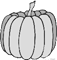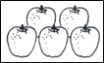• 题型：作图题 难易度：中等

3 .

看图圈数。• 题型：填空题 难易度：中等

4 .

○ ○ ○ ○ ○

☆ ☆ ☆ ☆ ☆ ☆

○比☆少（_______）个。

• 题型：填空题 难易度：中等

5 .

○ ○ ○ ○ ○

□ □ □ □

○比□多（_____）个

• 题型：填空题 难易度：中等

6 .

分一分。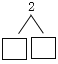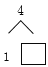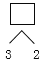• 题型：填空题 难易度：中等

7 .

在○里填上“>”、“<”或“=”。

4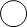1     34     155     42     34

• 题型：排序题 难易度：中等

8 .

把2、4、3、5、1、0这几个数按从大到小排：>>>>>• 题型：连线题 难易度：中等

9 .

把同样多的连一连。• 题型：解答题 难易度：中等

10 .（1）一共有（    ）只动物。

（2）把左边的3只动物圈起来。

（3）从左边数起，小鸡排第（   ），它的左边有（   ）只动物。

• 题型：计算题 难易度：中等

11 .

看图填一填。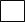+=• 题型：计算题 难易度：中等

12 .

看图填一填。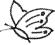+=• 题型：解答题 难易度：中等

13 .

把减掉的划去，再填一填。

3－1＝（   ）                5－2＝（   ）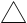• 题型：计算题 难易度：中等

14 .

看图列算式：• 题型：填空题 难易度：中等

15 .

妈妈把一根绳子剪了1次，剪成2段，那么剪2次剪成（_____）段。

• 下载word版试卷(含答案解析)